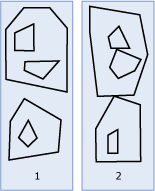# MultiPolygon

Applies to:SQL ServerAzure SQL DatabaseAzure SQL Managed Instance

A MultiPolygon instance is a collection of zero or more Polygon instances.

## Polygon Instances

The illustration below shows examples of MultiPolygon instances.As shown in the illustration:

• Figure 1 is a MultiPolygon instance with two Polygon elements. The boundary is defined by the two exterior rings and the three interior rings.

• Figure 2 is a MultiPolygon instance with two Polygon elements. The boundary is defined by the two exterior rings and the three interior rings. The two Polygon elements intersect at a tangent point.

### Accepted Instances

A MultiPolygon instance is accepted one of the following conditions is met.

• It is an empty MultiPolygon instance.

• All instances comprising the MultiPolygon instance are accepted Polygon instances. For more information on accepted Polygon instances, see Polygon.

The following examples show accepted MultiPolygon instances.

``````DECLARE @g1 geometry = 'MULTIPOLYGON EMPTY';
DECLARE @g2 geometry = 'MULTIPOLYGON(((1 1, 1 -1, -1 -1, -1 1, 1 1)),((1 1, 3 1, 3 3, 1 3, 1 1)))';
DECLARE @g3 geometry = 'MULTIPOLYGON(((2 2, 2 -2, -2 -2, -2 2, 2 2)),((1 1, 3 1, 3 3, 1 3, 1 1)))';
``````

The following example shows a MultiPolygon instance that will throw a `System.FormatException`.

``````DECLARE @g geometry = 'MULTIPOLYGON(((1 1, 1 -1, -1 -1, -1 1, 1 1)),((1 1, 3 1, 3 3)))';
``````

The second instance in the MultiPolygon is a LineString instance and not an accepted Polygon instance.

### Valid Instances

A MultiPolygon instance is valid if it is an empty MultiPolygon instance or if it meets the following criteria.

1. All of the instances comprising the MultiPolygon instance are valid Polygon instances. For valid Polygon instances, see Polygon.

2. None of the Polygon instances comprising the MultiPolygon instance overlap.

The following example shows two valid MultiPolygon instances and one invalid MultiPolygon instance.

``````DECLARE @g1 geometry = 'MULTIPOLYGON EMPTY';
DECLARE @g2 geometry = 'MULTIPOLYGON(((1 1, 1 -1, -1 -1, -1 1, 1 1)),((1 1, 3 1, 3 3, 1 3, 1 1)))';
DECLARE @g3 geometry = 'MULTIPOLYGON(((2 2, 2 -2, -2 -2, -2 2, 2 2)),((1 1, 3 1, 3 3, 1 3, 1 1)))';
SELECT @g1.STIsValid(), @g2.STIsValid(), @g3.STIsValid();
``````

`@g2` is valid because the two Polygon instances touch only at a tangent point. `@g3` is not valid because the interiors of the two Polygon instances overlap each other.

## Examples

### Example A.

The following example shows the creation of a `geometry``MultiPolygon` instance and returns the Well-Known Text (WKT) of the second component.

``````DECLARE @g geometry;
SET @g = geometry::Parse('MULTIPOLYGON(((0 0, 0 3, 3 3, 3 0, 0 0), (1 1, 1 2, 2 1, 1 1)), ((9 9, 9 10, 10 9, 9 9)))');
SELECT @g.STGeometryN(2).STAsText();
``````

## Example B.

This example instantiates an empty `MultiPolygon` instance.

``````DECLARE @g geometry;
SET @g = geometry::Parse('MULTIPOLYGON EMPTY');
``````